Urgent & Immediate-Proof of Vector Identity Using Tensors

Homework Statement

Hello everyone, can anyone help me prove this using tensors?
Given three arbitrary vectors not on the same line, A, B, C, any other vector D can be expressed in terms of these as: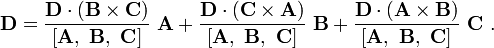where [A, B, C] is the scalar triple product A · (B × C)

Homework Equations

I know that scalar triple product A · (B × C) in tensor notation is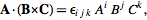and triple product can be written as: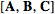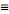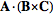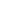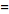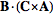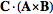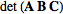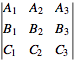The Attempt at a Solution

I just tried to prove as normal, and after simplifying numerator of fractions with their repective denominator in tensor form, finally I arrived to D=3D !!
thanks

Orodruin
Staff Emeritus
Homework Helper
Gold Member
2021 Award
You need to show us your actual attempt and not just describe it and give your result. If you do not show us what you did, how are we supposed to see where you went wrong and help you correct it?

We assume vectors have the following tensor notation: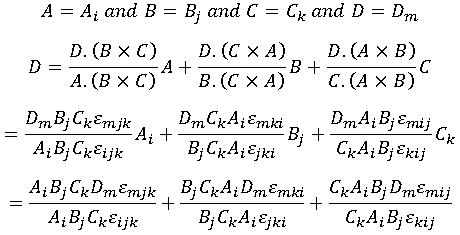After simplifying numerators with denominators we have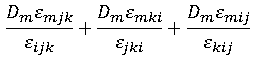And we know that the permutation symbol has the following characteristic (I also mentioned on vector algebra):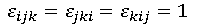So we have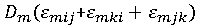And I assumed that (from permutation symbol characteristics):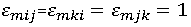So I arrived to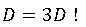Which is WRONG!

Last edited:
Orodruin
Staff Emeritus
Homework Helper
Gold Member
2021 Award
You cannot simply cancel terms in the denominator and numerator like you are doing. The repeated indices represent sums (Einstein's summation convention) and both the denominator and numerator are therefore containing sums where all of ##A_i## (for example) represent different values for each term of the sum. In other words, what you are doing is the equivalent of
$$\frac{x_1 y_1 + x_2 y_2}{x_1 z_1 + x_2 z_2} = \frac{y_1 + y_2}{z_1 + z_2}.$$
This is obviously not true unless you have very particular values for the numbers included.

And I assumed that (from permutation symbol characteristics):This is utter nonsense. The different ##\varepsilon##s do not even have the same indices so you cannot possibly expect this relation to hold.

You are also doing something obscure when you are trying to identify a vector with its components through ##A = A_i##. This cannot possibly hold as the left hand side is a vector and the right hand side is a number. You can take the component of a vector in a certain direction, but then you need to do that in the same direction for all terms of the vector. What you have done here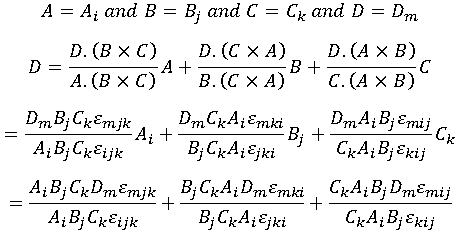between the second and third line does not make any sense. You should be taking the ##i##th component of the vector ##D##, which in the first term would be proportional to ##A_i##, in the second proportional to ##B_i##, and in the third to ##C_i## (note the same index ##i## on all of these). Until you have these basics down, you will have no chance of solving this problem.

Hello
the first note is true,
$$\frac{x_1 y_1 + x_2 y_2}{x_1 z_1 + x_2 z_2} = \frac{y_1 + y_2}{z_1 + z_2}.$$
is totally wrong and now I understood.
For your second note, I used the following: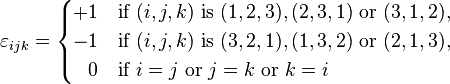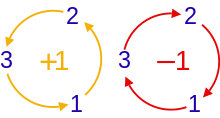and I just assumed m is replaced insted of k, j, i respectively.
whats the problem with it?
And for the third, I think I understood what you mean. I try to rewrite the answer and post it again.
thanks so much
I am beginner and the way I tried to prove is what my instructor used. I will try to correct it

Last edited:
Ray Vickson
Homework Helper
Dearly Missed
Hello
the first note is true,
$$\frac{x_1 y_1 + x_2 y_2}{x_1 z_1 + x_2 z_2} = \frac{y_1 + y_2}{z_1 + z_2}.$$
is totally wrong and now I understood.
For your second note, I used the following: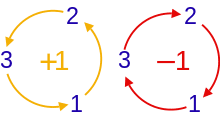and I just assumed m is replaced insted of k, j, i respectively.
whats the problem with it?
And for the third, I think I understood what you mean. I try to rewrite the answer and post it again.
thanks so much
I am beginner and the way I tried to prove is what my instructor used. I will try to correct it

You are doing it the hard way. If I were doing it I would just express ##\vec{D}## in the form ##\vec{D} = a \vec{A} + b \vec{B} + c \vec{C}##, then solve the equations for ##a,b,c##.

Orodruin
Staff Emeritus
Homework Helper
Gold Member
2021 Award
whats the problem with it?
All equations on index form must have the same indices on both sides, you cannot just assume that one index is replacable by another. ##\varepsilon_{ijk}## is only equal to ##\varepsilon_{mjk}## if ##i=m##, which is not always the case in your equations.

Orodruin
Staff Emeritus